International
Tables for
Crystallography
Volume B
Reciprocal space
Edited by U. Shmueli

International Tables for Crystallography (2010). Vol. B, ch. 2.5, pp. 366-367   | 1 | 2 |

## Section 2.5.6.1. The object and its projection

B. K. Vainshteinc and P. A. Penczekg

#### 2.5.6.1. The object and its projection

| top | pdf |

In electron microscopy (EM) and single-particle reconstruction, three-dimensional (3D) reconstruction methods are used for studying biological structures; that is, symmetric or asymmetric associations of biomacromolecules (muscles, spherical and rod-like viruses, bacteriophages, individual proteins and ribosomes) (Frank, 2006). The electron microscope is used to obtain parallel-beam two-dimensional (2D) projections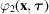(ray transform) of frozen hydrated 3D macromolecules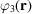suspended in random orientations (Fig. 2.5.6.1). The function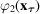is the 2D projection of the 3D molecular electron distribution. One can also consider one-dimensional (1D) projections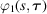of multidimensional distributions; the set of these projections is called a Radon transform. For 2D distributions, ray and Radon transforms differ only in the notation. For (d > 2)-dimensional distributions the two are different: in a ray transform the integrals are calculated over straight lines and yield (d − 1)-dimensional projections, while in Radon transforms the integrals are calculated over (d − 1)-dimensional hyperplanes and yield 1D projections. In electron microscopy, Radon transforms are not directly measurable, but can be formed computationally and used in intermediate steps of the 3D reconstruction or in alignment procedures (Radermacher, 1994).Figure 2.5.6.1 | top | pdf |A three-dimensional objectand its two-dimensional projection. The electron beam penetrates the specimen in the direction of the z axis.

Within the linear weak-phase-object approximation of the image-formation process in the microscope [see equation (2.5.2.42)in Section 2.5.2of this chapter], 2D projections represent line integrals of the potential of the particle under examination convoluted with the point-spread function of the microscope, s, so, using (2.5.2.43),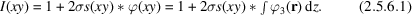Since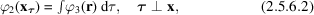we have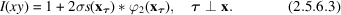If we omit constant terms, we obtain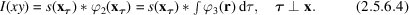In this section, we will assume that all images were collected using the same defocus setting, so the point-spread function s is constant and does not depend on the projection direction. Thus, we will concern ourselves with the inversion of the projection problem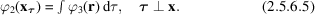The projection direction is defined by a unit vector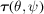and it is formed on the plane x perpendicular to. The set of various projections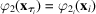may be assigned by a discrete or continuous set of points on a unit sphere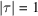(Fig. 2.5.6.2).Figure 2.5.6.2 | top | pdf |The projection sphere and projectionofalong τ onto the plane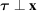. The case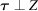represents orthoaxial projection. Points indicate an arbitrary distribution of projection directions τ.

In Fourier space, the relation between an object and its projection is referred to as the central section theorem: the Fourier transformation of projection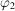of a 3D object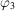is the central (i.e., passing though the origin of reciprocal space) 2D plane cross section of a 3D transform perpendicular to the projection vector (Bracewell, 1956; DeRosier & Klug, 1968; Crowther, Amos et al., 1970). In Cartesian coordinates, a 3D Fourier transform is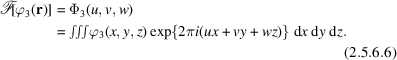The transform of projection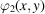along z is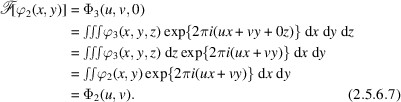In the general case of projecting along the vector, the central section theorem is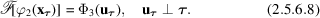From this theorem it follows that the inversion of the 3D ray transform is possible if there is a continuous set of projections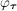corresponding to the motion of the vectorover any continuous line connecting the opposite points on the unit sphere (Fig. 2.5.6.2) (Orlov's condition: Orlov, 1976). This result is evidenced by the fact that in this case the cross sections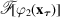that are perpendicular toin Fourier space continuously cover the whole Fourier space, i.e., they yield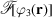and thereby determine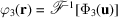.

In single-particle reconstruction, imaged objects are randomly and nonuniformly oriented on the substrate at different angles (Frank, 2006) and the distribution of their orientations is beyond our control; therefore, the practical impact of Orlov's condition is limited. In fact, it is more useful to determine a posteriori, i.e., after the 3D reconstruction of the macromolecule is computed, how well the Fourier space was covered. This can be done by calculating the distribution of the 3D spectral signal-to-noise ratio (Penczek, 2002).

### References

Bracewell, R. N. (1956). Strip integration in radio astronomy. Austr. J. Phys. 9, 198–217.
Crowther, R. A., Amos, L. A., Finch, J. T., DeRosier, D. J. & Klug, A. (1970). Three dimensional reconstruction of spherical viruses by Fourier synthesis from electron micrographs. Nature (London), 226, 421–425.
DeRosier, D. J. & Klug, A. (1968). Reconstruction of three dimensional structures from electron micrographs. Nature (London), 217, 130–134.
Frank, J. (2006). Three-Dimensional Electron Microscopy of Macromolecular Assemblies. New York: Oxford University Press.
Orlov, S. S. (1976). Theory of three-dimensional reconstruction 1. Conditions for a complete set of projections. Sov. Phys. Crystallogr. 20, 312–314.
Penczek, P. A. (2002). Three-dimensional spectral signal-to-noise ratio for a class of reconstruction algorithms. J. Struct. Biol. 138, 34–46.
Radermacher, M. (1994). Three-dimensional reconstruction from random projections: orientational alignment via Radon transforms. Ultramicroscopy, 53, 121–136.